اولين سايت تخصصي آموزش و نگهداري و تعميرات ديگ بخار، ديگ آبگرم، ديگ روغن داغ و آموزش نگهداري و طراحي تاسيسات در ايران به صورت کاملا فارسي، و با استناد به آخرين دستاوردهي تکنولوژي ديگ هاي بخار و تاسيسات مربوطه، در جهان.:::| درج آگهي | فروشگاه | | درباره ما | تماس با ما |:::

Convection Section Design:

In the convection section, heat is transferred by both radiation and convection. The convection transfer coefficients for fin and stud tubes are explored here as well as bare tube transfer. The short beam radiation is treated separately from the convection transfer below.
This section of Fired Heater Design is divided into five main areas, which can be selected from the subject drop down box above or you may use the jump links below to go to a section. You may also use your browser "back button" to return to where you were.

Overall Heat Transfer Coefficient, Uo:

Uo = 1/Rto

Where,

 Uo = Overall heat transfer coefficient, Btu/hr-ft2-F Rto = Total outside thermal resistance, hr-ft2-F/Btu

And,

 Rto = Ro + Rwo + Rio Ro = Outside thermal resistance, hr-ft2-F/Btu Rwo = Tube wall thermal resistance, hr-ft2-F/Btu Rio = Inside thermal resistance, hr-ft2-F/Btu

And the resistances are computed as,

 Ro = 1/he Rwo = (tw/12*kw)(Ao/Aw) Rio = ((1/hi)+Rfi)(Ao/Ai)

Where,

 he = Effective outside heat transfer coefficient, Btu/hr-ft2-F hi = Inside film heat transfer coefficient, Btu/hr-ft2-F tw = Tubewall thickness, in kw = Tube wall thermal conductivity, Btu/hr-ft-F Ao = Outside tube surface area, ft2/ft Aw = Mean area of tube wall, ft2/ft Ai = Inside tube surface area, ft2/ft Rfi = Inside fouling resistance, hr-ft2-F/Btu

Inside film heat transfer coefficient, hi:
The inside heat transfer coefficient calculation procedure is covered in detail, elsewhere in this course.

Effective outside heat transfer coefficient, he

he = 1/(1/(hc+hr)+Rfo)

Where,

 hc = Outside heat transfer coefficient, Btu/hr-ft2-F hr = Outside radiation heat transfer coefficient, Btu/hr-ft2-F Rfo = Outside fouling resistance, hr-ft2-F/Btu

Outside film heat transfer coefficient, hc:
The bare tube heat transfer film coefficient, hc, can be described by the following equations.
For a staggered tube arrangement,

hc = 0.33*kb(12/do)((cp*mb)/kb)1/3((do/12)(Gn/mb)))0.6

And for an inline tube arrangement,

hc = 0.26*kb(12/do)((cp*mb)/kb)1/3((do/12)(Gn/mb)))0.6

Where,

 hc = Convection heat transfer coefficient, Btu/hr-ft2-F do = Tube outside diameter, in kb = Gas thermal conductivity, Btu/hr-ft-F cp = Gas heat capacity, Btu/lb-F mb = Gas dynamic viscosity, lb/hr-ft Gn = Mass velocity of gas, lb/hr-ft2

We can describe a sample bare tube bank as follows:

 Process Conditions:Gas flow, lb/hr = 100,000Gas temperature in, °F = 1000Gas temperature out, °F = 868Compostion, molesN2, % = 71.5779O2, % = 2.8800CO2, % = 8.6404H2O, % = 16.4044Ar, % = 0.8609Mechanical Conditions:Tube Diameter, in = 4.500Tube Spacing, in = 8Number Tubes Wide = 8Tube Effective Length, ft = 13.000Number Of Tubes = 48Tube Arrangement = Staggered Pitch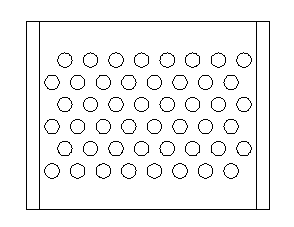Gas Properties
For the gas properties, we can use the script we used in the radiant section design to get the properties of the gas at the average temperature.

From this program, we get the following properties,
kb, Btu/hr-ft-F = 0.0315
cp, Btu/lb-F = 0.2909
mb, cp = 0.0340 = 0.0340*2.42 = 0.0823 lb/hr-ft
To calculate the mass velocity, Gn, we need to first calculate the net free area of the tube bank. For these calculations, we are going to assume the tube rows are corbelled, so the net free area, NFA:
NFA = Nwide*tspc/12*tlgth-Nwide*tOd/12*tlgth = 8*8/12*13-8*4.5/12*13 = 30.333 ft2
Therefore,
Gn = Wgas / NFA = 100000/30.333 = 3296.739
And using our formula for hc,

hc = 0.33*0.0315 (12/4.5)((0.2909 *0.0823 )/0.0315 )1/3((4.5/12)(3296.739/0.0823 )))0.6 = 8.1115

To get a feel for the values of the coeffcient, use the following script to run various designs.

 Tube Diameter, in: Tube Spacing, in: Number Tubes Wide: Tube Layout: Staggered Inline Tube Eff. Length, ft: Gas Flow, lb/hr: Gas Visc, lb/hr-ft: Therm.Cond., Btu/hr-ft-F: Spec. Heat, Btu/lb-F:

 Outside Film Coefficient, Btu/hr-ft2-F: Net Free Area, ft2: Mass Velocity, lb/hr-ft2:

The radiation transfer coefficient, hr is described later in this section. Fouling resistances, Rfi and Rfo are allowances that depend upon the process or service of the heater and the fuels that are being burned.

Convection Transfer, Fin Tubes

You will notice that the heat transfer equations for the fin tubes are basically the same as for the bare tubes untill you reach the he factor, where a new concept is introduced to account for the fin or extended surface. The procedure presented herein are taken from the Escoa manual which can be downloaded in full from the internet.

Overall Heat Transfer Coefficient, Uo:

Uo = 1/Rto

Where,

 Uo = Overall heat transfer coefficient, Btu/hr-ft2-F Rto = Total outside thermal resistance, hr-ft2-F/Btu

And,

 Rto = Ro + Rwo + Rio Ro = Outside thermal resistance, hr-ft2-F/Btu Rwo = Tube wall thermal resistance, hr-ft2-F/Btu Rio = Inside thermal resistance, hr-ft2-F/Btu

And the resistances are computed as,

 Ro = 1/he Rwo = (tw/12*kw)(Ao/Aw) Rio = ((1/hi)+Rfi)(Ao/Ai)

Where,

 he = Effective outside heat transfer coefficient, Btu/hr-ft2-F hi = Inside film heat transfer coefficient, Btu/hr-ft2-F tw = Tubewall thickness, in kw = Tube wall thermal conductivity, Btu/hr-ft-F Ao = Total outside surface area, ft2/ft Aw = Mean area of tube wall, ft2/ft Ai = Inside tube surface area, ft2/ft Rfi = Inside fouling resistance, hr-ft2-F/Btu

Inside film heat transfer coefficient, hi:
The inside heat transfer coefficient calculation procedure is covered in detail, elsewhere in this course.
Effective outside heat transfer coefficient, he:

he = ho(E*Afo+Apo)/Ao

Where,

 ho = Average outside heat transfer coefficient, Btu/hr-ft2-F E = Fin efficiency Ao = Total outside surface area, ft2/ft Afo = Fin outside surface area, ft2/ft Apo = Outside tube surface area, ft2/ft

And,
Average outside heat transfer coefficient, ho:

ho = 1/(1/(hc+hr)+Rfo)

Where,

 hc = Outside heat transfer coefficient, Btu/hr-ft2-F hr = Outside radiation heat transfer coefficient, Btu/hr-ft2-F Rfo = Outside fouling resistance, hr-ft2-F/Btu

Outside film heat transfer coefficient, hc:

hc = j*Gn*cp(kb/(cp*mb))0.67

Where,

 j = Colburn heat transfer factor Gn = Mass velocity based on net free area, lb/hr-ft2 cp = Heat capacity, Btu/lb-F kb = Gas thermal conductivity, Btu/hr-ft-F mb = Gas dynamic viscosity, lb/hr-ft

Colburn heat transfer factor, j:

j = C1*C3*C5(df/do)0.5((Tb+460)/(Ts+460))0.25

Where,

 C1 = Reynolds number correction C3 = Geometry correction C5 = Non-equilateral & row correction df = Outside diameter of fin, in do = Outside diameter of tube, in Tb = Average gas temperature, F Ts = Average fin temperature, F

Reynolds number correction, C1:

C1 = 0.25*Re-0.35

Where,

 Re = Reynolds number

Geometry correction, C3:
For segmented fin tubes arranged in, a staggered pattern

C3 = 0.55+0.45*e(-0.35*lf/Sf)

an inline pattern,

C3 = 0.35+0.50*e(-0.35*lf/Sf)

For solid fin tubes arranged in, a staggered pattern

C3 = 0.35+0.65*e(-0.25*lf/Sf)

an inline pattern

C3 = 0.20+0.65*e(-0.25*lf/Sf)

Where,

 lf = Fin height, in sf = Fin spacing, in

Non-equilateral & row correction, C5:
For fin tubes arranged in, a staggered pattern

C5 = 0.7+(0.70-0.8*e(-0.15*Nr^2))*e(-1.0*Pl/Pt)

an inline pattern

C5 = 1.1+(0.75-1.5*e(-0.70*Nr^2))*e(-2.0*Pl/Pt)

Where,

 Nr = Number of tube rows Pl = Longitudinal tube pitch, in Pt = Transverse tube pitch, in

Mass Velocity, Gn:

Gn = Wg/An

Where,

 Wg = Mass gas flow, lb/hr An = Net free area, ft2

Net Free Area, An:

An = Ad - Ac * Le * Nt

Where,

 Ad = Cross sectional area of box, ft2 Ac = Fin tube cross sectional area/ft, ft2/ft Le = Effective tube length, ft Nt = Number tubes wide And, Ad = Nt * Le * Pt / 12 Ac = (do + 2 * lf * tf * nf) / 12 tf = fin thickness, in nf = number of fins, fins/in

Surface Area Calculations:
For the prime tube,

Apo = Pi * do (1- nf * tf) / 12

And for solid fins,

Ao = Pi*do(1-nf* tf)/12+Pi*nf(2*lf(do+lf)+tf(do+2*lf))/12

And for segmented fins,

Ao = Pi*do(1-nf* tf)/12+0.4*Pi*nf(do+0.2)/12+Pi*nf (do+0.2)((2*lf-0.4)(wn+tf)+ws*tf)/(12*ws)

And then,

Afo = Ao - Apo

Where,

 ws = Width of fin segment, in

We can describe a sample fin tube bank as follows:

 Process Conditions:Gas flow, lb/hr = 100,000Gas temperature in, °F = 1000Gas temperature out, °F = 591Average fin temperature, °F = 755Compostion, molesN2, % = 71.5779O2, % = 2.8800CO2, % = 8.6404H2O, % = 16.4044Ar, % = 0.8609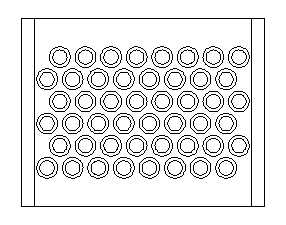Mechanical Conditions:Tube Diameter, in = 4.500Tube Spacing, in = 8Number Tubes Wide = 8Tube Effective Length, ft = 13.000Number Of Tubes = 48 Tube Arrangement = Staggered PitchFin Height, in = 0.75Fin Thickness, in = 0.05Fin Density, fins/in = 6Fin Type = SegmentedFin Segment Width, in = 0.3125

Gas Properties
For the gas properties, we can use the script we used in the radiant section design to get the properties of the gas at the average temperature. From this program, we get the following properties,
kb, Btu/hr-ft-F = 0.0290
cp, Btu/lb-F = 0.2858
mb, cp = 0.0317 = 0.0317*2.42 = 0.0767 lb/hr-ft
To calculate the mass velocity, Gn, we need to first calculate the net free area of the tube bank. For these calculations, we are going to assume the tube rows are corbelled, so the net free area, An:
Ac = (4.5+2*0.75*0.05*6)/12 = 0.4125 So,

An = 69.333 - 0.4125 * 13 * 8 = 26.4333

And,

Gn = 100000 / 26.4330 = 3783.1069

Now we can calculate the reynolds number, Re,

Re = 3783.1069*4.5/(12*0.0767) = 18496.2854

And,

C1 = 0.25*18496.2854-0.35 = 0.0080

For, sf = 1/6-.05=0.1167

C3 = 0.55+0.45*e(-0.35*0.75/0.1167) = 0.5975

And,
Pl = (82-8/22)0.5=6.9282

C5 = 0.7+(0.70-0.8*e(-0.15*6^2))*e(-1.0*6.9282/8) = 0.9929

Now we can calculate the Colburn factor,

j = 0.0080*0.5975*0.9929(6/4.5)0.5((795.5+460)/(755+460))0.25 = 0.0055

And finally,

hc = 0.0055*3783.1069*0.2858 (0.0290 /(0.2858*0.0767))0.67 = 7.1732

To get a feel for the values of the coeffcient, use the following script to run various designs.

 Tube Diameter, in: Tube Spacing, in: Number Tubes Wide: Tube Layout: Staggered Inline Number Tube Rows: Tube Eff. Length, ft: Fin Height, in: Fin Density, fins/in: Fin Thickness, in: Fin Segment Width, in: Average Fin Temperature, F: Fin Type: Segmented Solid Gas Flow, lb/hr: Average Gas Temperature,F: Therm.Cond., Btu/hr-ft-F: Spec. Heat, Btu/lb-F: Gas Visc, lb/hr-ft:

 Outside Film Coefficient, Btu/hr-ft2-F: Net Free Area, ft2: Mass Velocity, lb/hr-ft2:

The radiation transfer coefficient, hr is described later in this section. Fouling resistances, Rfi and Rfo are allowances that depend upon the process or service of the heater and the fuels that are being burned.

Fin Efficiency, E:
For segmented fins,

E = x * (0.9 + 0.1 * x)

And for solid fins,

E = y * (0.45 * ln(df / do) * (y - 1) + 1)

Where,

y = x * (0.7 + 0.3 * x)

And,

x = tanh(m * B) / (m * B)

Where,

B = lf + (tf /2)

For segmented fins,

m = (ho (tf + ws) / (6 * kf * tf * ws))0.5

And for solid fins,

m = (ho / (6 * kf * tf))0.5

Fin Tip Temperature, Ts:
The average fin tip temperature is calculated as follows,

Ts = Tg + (Tw - Tg) * 1/((e1.4142mB+e-1.4142mB)/2)

Maximum Fin Tip Temperature, Tfm:
The maximum fin tip temperature is calculated as follows,

Tsm = Twm + q(Tgm - Twm)

Where,

 Tsm = Maximum Fin Tip Temperature, F Tgm = Maximum Gas Temperature, F Twm = Maximum Tube Wall Temperature, F

And, The value for theta, q, can be described by the following curve.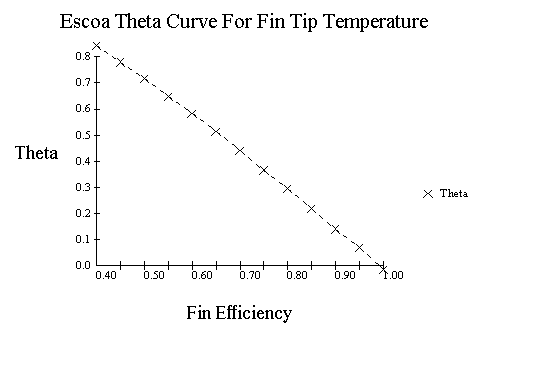Convection Transfer, Stud Tubes

For studded tubes, the correlations used are as provided by Birwelco, Ltd.

Overall Heat Transfer Coefficient, Uo:

Uo = 1/Rto

Where,

 Uo = Overall heat transfer coefficient, Btu/hr-ft2-F Rto = Total outside thermal resistance, hr-ft2-F/Btu

And,

 Rto = Ro + Rwo + Rio Ro = Outside thermal resistance, hr-ft2-F/Btu Rwo = Tube wall thermal resistance, hr-ft2-F/Btu Rio = Inside thermal resistance, hr-ft2-F/Btu

And the resistances are computed as,

 Ro = 1/he Rwo = (tw/(12*kw))(Ao/Aw) Rio = ((1/hi)+Rfi)(Ao/Ai)

Where,

 he = Effective outside heat transfer coefficient, Btu/hr-ft2-F hi = Inside film heat transfer coefficient, Btu/hr-ft2-F tw = Tubewall thickness, in kw = Tube wall thermal conductivity, Btu/hr-ft-F Ao = Outside surface area, ft2/ft Aw = Mean area of tube wall, ft2/ft Ai = Inside tube surface area, ft2/ft Rfi = Inside fouling resistance, hr-ft2-F/Btu

Effective outside heat transfer coefficient, he: For staggered and inline pitch,

he = (hso*E*Afo+ht*Apo)/Ao

Where,

 ht = Base tube outside heat transfer coefficient, Btu/hr-ft2-F hso = Stud outside heat transfer coefficient, Btu/hr-ft2-F Ao = Total outside surface area, ft2/ft Afo = Stud outside surface area, ft2/ft Apo = Tube outside surface area, ft2/ft

Inline pitch correction,

he = he*(do/Pl)0.333

Where,

 do = Outside tube diameter, in Pl = Longitudinal pitch of tubes, in

Base tube outside heat transfer coefficient, ht:

ht = (0.717/do0.333)(Gn/1000)0.67(Tb+460)0.3

And the stud coefficient,

hs = 0.936*(Gn/1000)0.67(Tb+460)0.3

With fouling,

hso = 1/(1/hs+Rfo)

Where,

 hs = Stud outside heat transfer coefficient, Btu/hr-ft2-F Gn = Mass velocity of flue gas, lb/hr-ft2 Tb = Average gas temperature, F

Stud efficiency, E:

E = 1/((ex+e-x)/1.950)

Where,

X = Ls/12((2*hso)/(ks*Ds/12))0.5

And,

 Ls = Length of stud, in Ds = Diameter of stud, in ks = Conductivity of stud, Btu/hr-ft-F

The following script will allow us calculate the coeffcient for stud tubes.

 Tube Diameter, in: Number Tubes Wide: Tube Longitudinal Pitch, in: Tube Transverse Pitch, in: Tube Layout: Staggered Inline Number Tube Rows: Tube Eff. Length, ft: Stud Length, in: Studs Per Plane: Stud Diameter, in: Planes Per Foot: Conductivity Of Stud, Btu/hr-ft-F: Outside Fouling Factor, hr-ft2-F/Btu: Gas Flow, lb/hr: Average Gas Temperature,F:

 Outside Film Coefficient, Btu/hr-ft2-F: Net Free Area, ft2: Mass Velocity, lb/hr-ft2:

The gas radiation factor, hr, can be calculated from the following correlations. This factor is used in calculating the overall heat transfer coefficient for bare tubes and fin tubes. The formulas for the stud tubes has this factor built into the equations. For bare tubes,

hr = 2.2*gr*(pp*mbl)0.50

And for fin tubes,

hr = 2.2*gr*(pp*mbl)0.50(Apo/Ao)0.75

Where,

 hr = Average outside radiation heat transfer coefficient, Btu/hr-ft2-F gr = Outside radiation factor, Btu/hr-ft2-F pp = Partial pressure of CO2 & H2O, , atm mbl = Mean beam length, ft Apo = Bare tube exposed surface area, ft2/ft Ao = Total outside surface area, ft2

Outside radiation factor, g r: The outside radiation factor can be described by the following curves: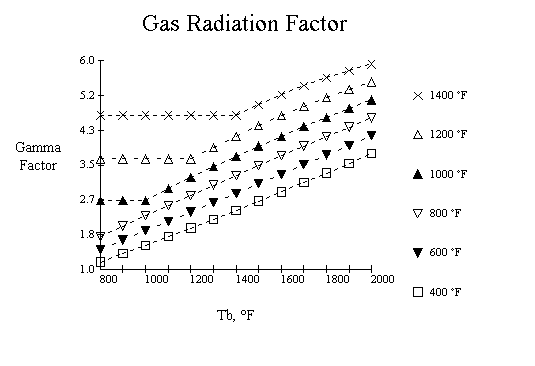»» جهت دریافت اطلاعات کامل در مورد این مقاله و پکیج محاسبات حرارتی با شماره تلفن 09126271381 تماس حاصل فرمایید.

 :::| صفحه اصلي | درج آگهي | فروشگاه | | درباره ما | تماس با ما |:::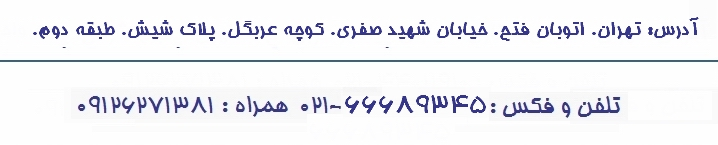©کليه حقوق مادي و معنوي اين سايت متعلق به این شرکت است. هر گونه کپي برداري از اطلاعات سايت بدون اطلاع مدير سايت ممنوع و ذکر تمامي مطالب با درج منبع بلامانع است.

منوی اصلی

لیست مقاله ها

لینک های ثابت

 واحد طراحی گروه مشاوره محصولات

اطلاع از به روز رسانی

 عضويت لغو عضويت

جستجو

 وب سایت